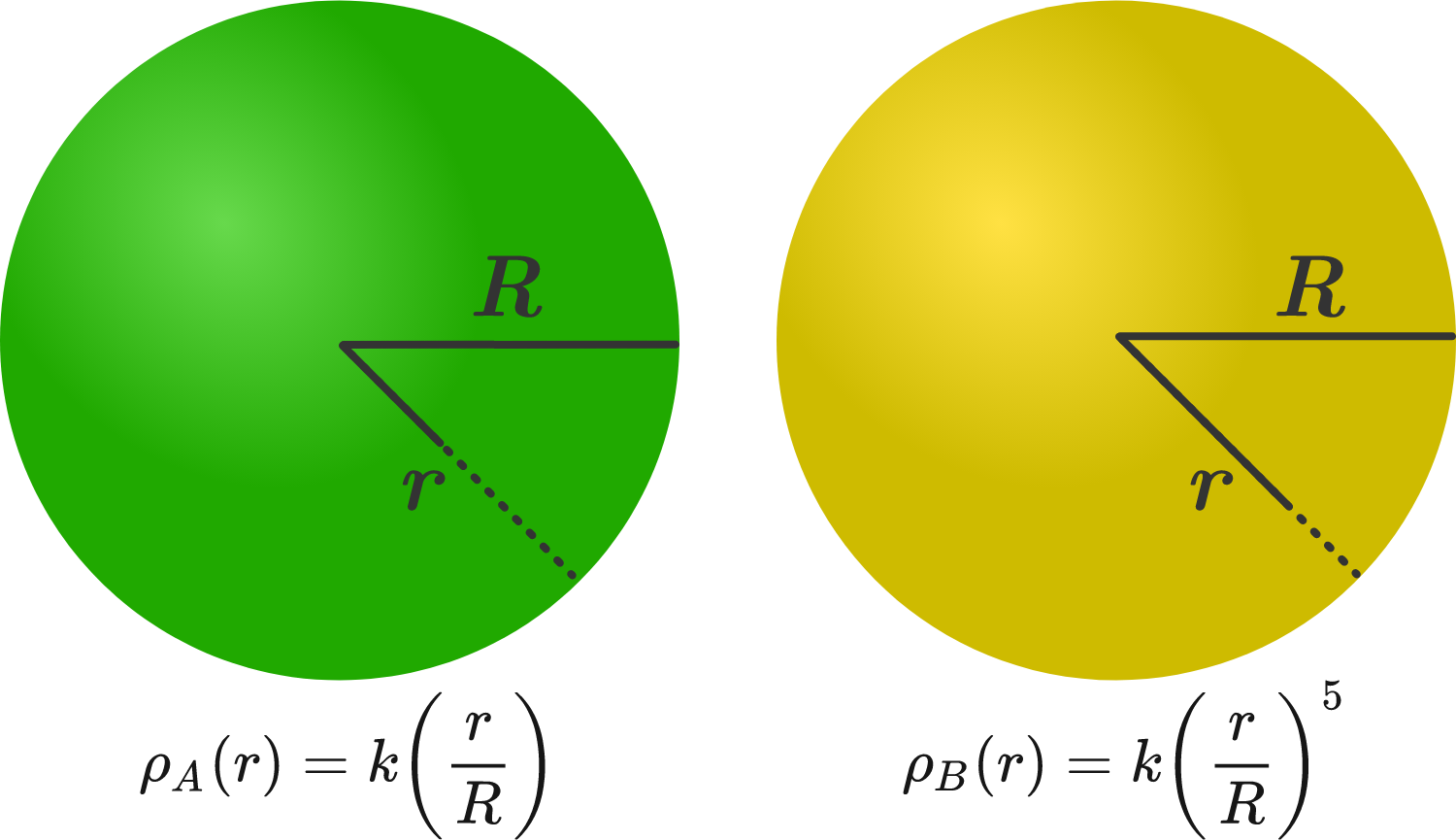# Rotating spheres of variable densities!

The densities of two solid spheres $A$ and $B$ of the same radius $R$ vary with radial distance $r$ as

$\rho_A (r) = k \left( \dfrac rR \right) \quad \text{and} \quad \rho_B (r) = k {\left( \dfrac rR \right)}^5,$

respectively, where $k$ is a constant.

Let the moments of inertia of the individual spheres about the axes passing through their centers be $I_A$ and $I_B$, respectively.

If $\ \dfrac{I_B}{I_A} = \dfrac{n}{10}$, then find the value of $n$.×